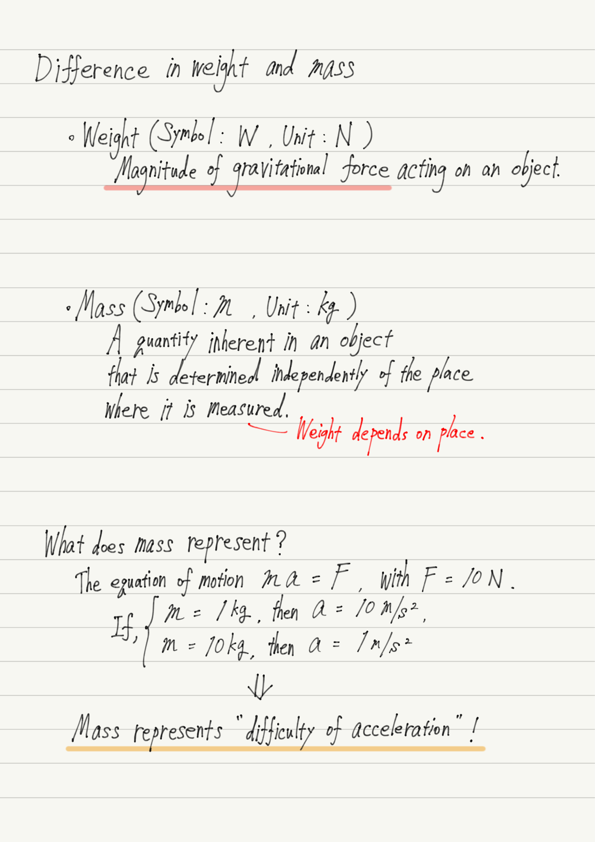# Weight and Mass

In the past, we learned how to calculate the magnitude of gravitational force, and then “mass” came into the picture.
However, there are quite a few people who confuse mass and weight.

“I know that weight and mass are two different concepts, but I don’t know exactly what the difference is…”

I have the impression that many people are like that.
In this lecture, you will understand the difference between weight and mass!

First, the question. Does body weight refer to weight? Or does it refer to mass?

Before we get to the answer, let’s first check the difference between weight and mass!

## Summary of this lecture## Focus on units of weight and mass

Now that you know the difference between weight and mass, let’s check your answers to the previous questions.

Body weight is “mass”!

As noted in the summary, weight is the magnitude of gravitational force.
For example, the moon has less gravity than the earth, so the weight measured on the moon will be smaller than the weight measured on the earth.

But just because you measured on the moon and the scale read smaller, this doesn’t mean you actually lost body weight!
It is just an “apparent” reduction.
A person’s body weight cannot be reduced just by changing the place.

Weight should be a person’s own inherent quantity that does not change no matter where it is measured.

It is also obvious when comparing the units.
The unit of mass is kg, whereas the unit of weight is N.
This shows that body weight is mass.

Weight and mass. Both of these words are commonly used in physics, so make sure you understand the difference between them and use them properly!

error: Content is protected !!